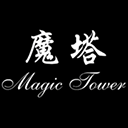# 经典魔塔50层苹果版 v1.1.7官方版

• 详情
• 相关
• 评论
•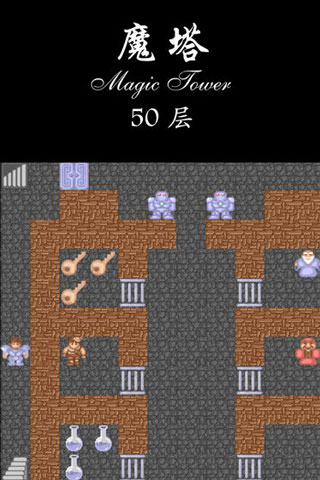•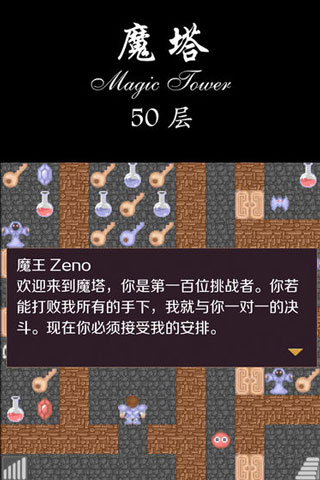•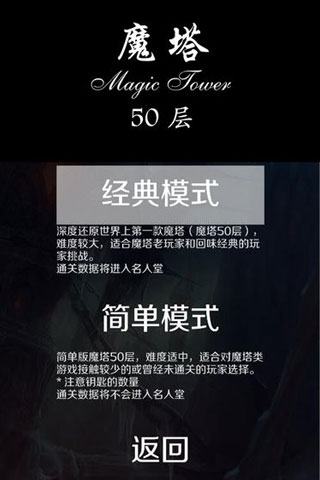••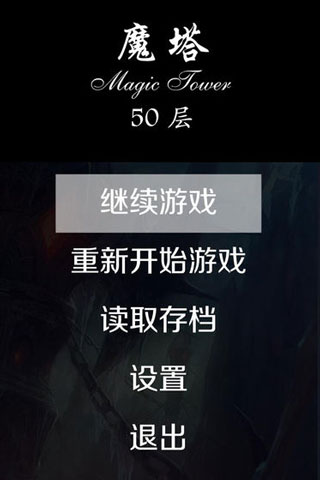1、经典复古像素风格打造
2、简单有趣游戏操作方式
3、丰富烧脑全新游戏关卡
4、全新魔性游戏玩法体验

### 经典魔塔50层攻略图鉴

1－10层：
1-2F：刚上来是按照预设剧情丢掉600血和兵器防具，行走路线只有一条，所以无话可说。
3F：和小偷一起越狱之后，直接上3F，再上中吃完瓶子和钥匙[L=1000，A=10，D=10，G=4]，向右打绿色史莱姆 [L=976，G=5]，找老头要手册，然后向下打红色史莱姆[L=926，G=7]，开门上楼。
4F：过黄门，直接向左，打绿色史莱姆[L=902，G=8]，打红色史莱姆[L=852，G=10]，开门上楼。
5F：向上打绿色史莱姆[L=828，G=11]，向右打绿色史莱姆[L=804，G=12]，向下打红色史莱姆[L=754，G=14]，开门拿剑[L=754，A=20，D=10，G=14]。向上打红色史莱姆[L=734，G=16]，不过门，回到中路打蝙蝠拿钥匙[L=678，G=19]，下楼。
4F：直接过黄门打红色史莱姆[L=658，G=21]，再过蓝门去商店，升级防御[L=658，A=20，D=14，G=1]。上楼。
5F：到右上角取钥匙，两个绿色史莱姆只打一个[L=654]。到中路过门打红色史莱姆[L=6 42]，打蝙蝠[L=594]，开门上楼。
6F：向下右转打红色史莱姆[L=582]，打初级法师[L=510]，拿钥匙，向上左转，进门打红色史莱姆[L=498]拿钥匙，然后向下过两个门，打红色史莱姆[L=486]补血[L=586]，过门红色史莱姆[L=574]，上楼。
7F：向上出门，左转走到初级法师面前向下，打蝙蝠拿钥匙[L=526]，打初级法师[L=454]，打骷髅战士[L=226]，开门上楼。
8F：向下开门吃瓶[L=276]打红/绿/红色史莱姆[L=248]，向下开门右转打骷髅[L=16 4]，向上打蝙蝠[L=116]，再向上打初级法师[L=44]，拿钥匙开门上楼。
9F：向右开门打绿色史莱姆[L=40]，吃瓶[L=90]，向下走到两扇门前再向下，从漏洞取盾[L=90，A=20，D=24，G=56]，回头一直下到5楼。
5F：过门向下打蝙蝠[L=62]，拿两把钥匙，再回到9楼。
9F：过两个黄门拿钥匙和红宝石[L=62，A=21，D=24，G=59]，再向左过黄门，打蝙蝠[L=48]，拿钥匙和蓝宝石[L=48，A=21，D=25，G=62]，回头一直下到7楼。
7F：在上楼梯边上那一竖列，开门，打蝙蝠[L=35]，吃瓶拿红宝石[L=85，A=22，D=25，G=65]，然后一直下到3楼。
3F：去左上角，打蝙蝠[L=72]，向上过门打初级法师[L=44]，拿钥匙、蓝宝石和血瓶[L=94，A=22，D=26，G=73]，然后上4楼。
4F：去左上角，打初级法师[L=70]，吃血拿黄/蓝钥匙[L=120]，上8楼。
8F：去左下角打蝙蝠[L=108]，过黄门打绿色史莱姆[不伤]，打蝙蝠[L=96]，过蓝门拿钥匙和红/蓝宝石[L=96，A=23，D=27，G=85]，下到1楼。
1F：去右中附近，过黄门打蝙蝠[L=85]，打初级法师[L=70]，打蝙蝠[L=59]，进黄门拿钥匙、血瓶和红/蓝宝石[L=109，A=24，D=28，G=96]，然后去4楼。
4F：去左下过黄门，打蝙蝠[L=99]拿2把钥匙，去6楼。
6F：去左下打骷髅[L=71]，打绿色史莱姆[不伤]，过黄门打蝙蝠[L=61]，拿蓝宝石并和老头说话[L=61，A=24，D=29，G=109]，回头下到3楼。
3F：去左中过黄门打骷髅[L=35]，拿钥匙、血瓶和红宝石[L=85，A=25，D=29，G=115]，上到4楼。
4F：下中偏右，过黄门打初级法师[L=76]，拿红宝石和血瓶[L=126，A=26，D=29，G=120]，上到5楼。
5F：左下方向打骷髅战士[L=57]，拿钥匙、蓝宝石、血瓶和记事本[L=107，A=26，D=30，G=128]，然后下到4楼。
4F：到左下打绿色史莱姆[不伤]，拿钥匙，下到1楼。
1F：左中方向过黄门吃瓶打骷髅[L=133]，向下过门拿钥匙打骷髅战士[L=67]，过黄门拿血瓶[L=167]、升降梯和钥匙，然后上到7楼。
7F：右数第2列完全打通，向上打骷髅[L=143]，拿血瓶和钥匙[L=193]，向下打骷髅战士[L=127]，拿血瓶和钥匙[L=327]，然后上到9楼。
9F：沿路向下中走，打红色史莱姆[不伤]，打蝙蝠[L=319]，过黄门向左，向上打骷髅
[L=295]，过黄门拿钥匙打骷髅战士[L=229]，过黄门拿钥匙打骷髅战士[L=163]，过黄门拿血瓶上楼。
10F：过黄门打骷髅[L=189]，拿蓝宝石[L=189，A=26，D=31，G=190]，向下过黄门打两个初级法师[L=183]，过黄门打骷髅[L=161]，拿红宝石[L=161，A=27，D=31，G=206]。到此为止，第一关升级完成，下面是补点血准备过关了。过关需要530的血[6个骷髅+2个骷髅战士+骷髅队长=6X22+2X63+272=530]。所以先去找血，下到9楼。
9F：右下角过黄门打初级法师[L=158]，拿血瓶和钥匙[L=208]，然后下到4楼。
4F：去右上打骷髅[L=186]，过黄门打骷髅战士[L=123]，拿血瓶和钥匙[L=323]，和老头说话，然后上到8楼。
8F：右中偏下打初级法师[L=320]，过黄门打两个初级卫兵[L=14]，拿血瓶和钥匙[L=26 4]，然后下到7楼。
7F：右数第3列下方，过黄门打红色史莱姆[不伤]，打初级法师[L=261]，拿血瓶[L=461]，然后下到1楼。
1F：右下角过黄门打蝙蝠[L=454]，拿血瓶[L=654]，然后上10楼。
10F：决斗开始，一场屠杀之后胜利[L=124]，拿战利品红/蓝宝石、钥匙和1个血瓶
[L=324，A=30，D=34，G=346]。

1F：绿色史莱姆X2
2F：老样子，没动
3F：初级法师X1，蝙蝠X1 。红瓶X2，黄钥匙X1。
4F：绿色史莱姆X2
5F：初级法师X1，绿色史莱姆X1
6F：骷髅战士X1，骷髅X2，初级法师X2，蝙蝠X1，绿色史莱姆X1； 红瓶X1，黄钥匙X1。
7F：骷髅战士X1，红色史莱姆X3，绿色史莱姆X4
8F：骷髅战士X1，骷髅X1，绿色史莱姆X1 ，蓝钥匙X1，黄钥匙X1，红瓶X1。 9F：骷髅战士X2，骷髅X2，初级法师X1，绿色史莱姆X1 ，黄钥匙X1。
10F：骷髅战士X2，骷髅X2； 蓝瓶X3。11－20层：
11F：直接向右，打蝙蝠上楼[L=320]。
12F：向左打一个蝙蝠[L=316]，升2次防1次攻[L=316，A=34，D=50，G=92]，打蝙蝠上楼[不伤]。
13F：直接上楼。
14F：过黄门，向左打大史莱姆[L=276]，打开下中的黄门，向下过漏洞，拿血瓶[L=376]。回到11层。
11F：走到去10层楼梯的左侧，进黄门，打大史莱姆[L=336]，回14楼直接上楼。 15F：向右过黄门，打蝙蝠[不伤]，向上过两道黄门，打大蝙蝠[L=236]，向右过黄门打大史莱姆[L=196]，和小偷说话，下到12层。
12F：吃掉商店门口两个血瓶[L=396]，在左下过黄门，打兽人[L=116]，拿钥匙，上到16层。
16F：拿钥匙，向下过蓝门，拿血瓶和钥匙[L=216]，向下过黄门，再向左过黄门，和老头说话，打大史莱姆[L=176]，向上过漏洞，拿钥匙、红/蓝宝石和血瓶[L=276，A=36，D=52，G=160]，上楼。
17F：向左打大蝙蝠[L=180]，拿血瓶[L=280]，过黄门，打4个初级卫兵[不伤]，拿剑
[L=280，A=56，D=52，G=220]，下到12层。
12F：升级攻击力[L=280，A=60，D=52，G=0]，下到8楼。
8F：去右下，过两道黄门，打骷髅[不伤]骷髅战士[不伤]，拿血瓶和钥匙[L=330]，回到11楼。
11F：向左下打大蝙蝠[L=282]，过蓝门拿血瓶[L=682]，打两个高级法师拿盾[L=424，A=60，D=72，G=70]，上到12层。
12F：到右下，打兽人[L=372]，过黄门打大史莱姆[不伤]，拿血瓶、钥匙和蓝宝石[L=472，A=60，D=74，G=96]，上到15层。
15F：到左上过黄门，打兽人拿蓝宝石[L=428，A=60，D=76，G=114]，下到11层。 11F：贴着右墙上去打大蝙蝠[L=404]，打大史莱姆[不伤]，拿血瓶和钥匙[L=504]，向左过黄门打兽人[L=468]，再过黄门拿红宝石[L=468，A=62，D=76，G=152]，下到6楼。
6F：打右上角的初级法师[不伤]，拿钥匙，上到12楼。
12F：从右侧往中间走过黄门，打高级法师[L=411]，拿血瓶[L=811]，向上打兽人[L=775]，过黄门，随便打一个高级法师[L=718]，拿钥匙和红宝石[L=718，A=6 4，D=76，G=219]，上到14楼。
14F：从右下打大史莱姆[不伤]，向上过黄门打兽人[L=682]，拿血瓶[L=782]，向左打大蝙蝠[L=758]，向上过黄门打兽人武士[L=494]，拿血瓶、钥匙和蓝宝石[L=594，A=6 4，D=78，G=287]，上到17楼。
17F：去右下打大蝙蝠[L=572]，拿血瓶[L=672]，向上过黄门打2个兽人[L=616]，再向上打2个兽人武士[L=112]，拿血瓶、钥匙和红/蓝宝石[L=512，A=66，D=80，G=395]，然后沿中间偏左的那条路，一路闯过[L=222]，上楼。
18F：向右过黄门打高级法师[L=192]，拿钥匙，在楼梯的正下方过漏洞，过蓝门，向左打兽人[L=172]，向下过黄门打高级法师[L=142]，拿血瓶、钥匙和红宝石[L=242，A=68，D=80，G=527]，下到16楼。
16F：右上过黄门打大史莱姆[不伤]，拿血瓶[L=342]，向下过黄门打兽人[L=322]，拿蓝钥匙，上到18楼。
18F：左上过蓝门和老头说话，上楼。
19F：向下过黄门打蝙蝠[不伤]，再向下过黄门打大蝙蝠[不伤]，向右打大史莱姆[不伤]，一直向右打兽人武士[L=82]，拿血瓶和钥匙[L=182]，在右下打大蝙蝠和蝙蝠[不伤]，过黄门拿钥匙，转身贴右墙向上打兽人[L=162]，过黄门打大史莱姆[不伤]，过黄门打大蝙蝠[不伤]，拿钥匙和红宝石[L=162，A=70，D=80，G=659]，下到6楼。
6F：到右上打绿色史莱姆[不伤]，过黄门打蝙蝠[不伤]，找商人花50元买蓝钥匙，拿血瓶[L=212]，上到9楼。
9F：去左上打骷髅战士[不伤]，拿钥匙，上到20楼。
20F：去左下打高级法师[L=182]，拿血瓶，向上过黄门打蝙蝠[不伤]，拿钥匙和红/蓝宝石[L=282，A=72，D=82，G=6 46]，下到18楼。
18F：去右下打石头人[L=210]，向下过黄门打高级法师[L=184]，拿血瓶、钥匙和蓝宝石[L=284，A=72，D=84，G=696]，下到16楼。
16F：去左上过黄门打大蝙蝠[不伤]，拿钥匙，下到15楼。
15F：贴左墙向下，过黄门打大史莱姆[不伤]，向右过黄门拿钥匙，上到19楼。
19F：到中间过蓝门向上打一个石头人，利用十字架墙那一横下面的漏洞，拿到十字架墙中心点藏的十字架[这下就不怕兽人武士了]，打左边的兽人武士[L=148]，拿血瓶[L=248]，打高级法师[L=226]，拿蓝钥匙，下到14层。
14F：从中间去左上角，打石头人[L=162]，打大蝙蝠[不伤]，拿血瓶[L=262]，过蓝门打3个兽人武士[L=46]，拿红钥匙，下到10层。
10F：把3个血瓶吃掉[L=6 46]，上到20楼。
20F：过红门，决战吸血鬼[L=71]，拿钥匙、红蓝宝石和1个血瓶[L=471，A=78，D=90，G=1070]，上楼。21-30层：
21F：和老头说话，上楼。
22F：上楼。
23F：把隐藏的砖头都撞出来，应该是“Go OF”的字样，和老头说话上楼。
24F：上楼。
25F：上楼。
26F：上楼。
27F：和老头说话上楼。这里注意，因为我们有很多瓶子没有吃，也没有尽情的升级攻防，钥匙都没有拿完，所以老头说的可以不听。
28F：上楼。这里的商人是100一枚回收黄钥匙的，到后面可以买些便宜的来倒货，不过肯定是不行了。
29F：和小偷说话，上楼。
30F：兵不血刃解决掉6个史莱姆，上楼。31-40层：
31F：向下干掉2个兽人武士[L=351]，上楼。
32F：刚上楼就被骑士队长攻击[L=51]，下到20楼。
20F：拿两个血瓶[L=851]，回到32楼。
32F：硬拼掉右下角的鬼战士[L=491]，去商店升级2次防御[L=491，A=78，D=122，G=527]，回到20楼。
20F：杀掉左下角的石头人，下到19楼。
19F：杀掉堵门的兽人武士，回到32楼。
32F：升级一次攻击[L=491，A=86，D=122，G=5]，从右上进黄门，打鬼战士[L=317]，进黄门拿钥匙和血瓶[L=1117]，上楼。
33F：过黄门拿血瓶[L=1917]，向下硬拼2个鬼战士和2个战士[L=165]，拿剑[L=165，A=126，D=122，G=200]，下到15楼。
15F：贴着右墙向下打高级法师[不伤]，拿血瓶[L=565]，回到32楼。
32F：从上中过黄门，打幽灵[L=511]，拿钥匙，拼战士[L=277]，开黄门拿红/蓝宝石
[L=277，A=130，D=126，G=297]，上楼。
33F：从上中过黄门，打幽灵[L=249]，拿血瓶和钥匙[L=449]，和老头说话，打兽人[不伤]，开黄门上楼。
34F：拿血瓶打幽灵[L=621]，过黄门向下，过黄门拼鬼战士[L=513]，过黄门向右再过黄门拼战士[L=291]，拿钥匙、血瓶和红宝石[L=491，A=134，D=126，G=467]，下到14楼。
14F：先到右上角打兽人[不伤]过黄门拿钥匙，再到左下角过黄门打高级法师[不伤]拿蓝钥匙，下到2楼。
2F：过蓝门硬拼两个中级卫兵[L=59]，拿钥匙和血瓶[L=659]，和右上老头说话，和小偷说话，回到32楼。
32F：升级一次攻击[L=659，A=142，D=126，G=879]，上到34楼。
34F：在上面向右过黄门，打幽灵[L=631]，拿钥匙拼骑士[L=215]，过黄门拿钥匙、血瓶和蓝宝石[L=415，A=142，D=130，G=974]，下到31楼
31F：向右打鬼战士[L=365]，向上过黄门打鬼战士[L=315]，和老头说话拿血瓶[L=515]，打战士[L=375]，过黄门拿红宝石[L=375，A=146，D=130，G=1089]，到右下过两道黄门拿血瓶[L=1175]，向左打鬼战士[L=1125]，过两道黄门拿蓝钥匙，上到35楼。
35F：从密道绕过龙，上楼。
36F：一路向下闯过去[L=255]，上楼。
37F：从下方一路闯过，拿血瓶[L=245]，下到12楼。
12F：去左上角，打高级法师[不伤]，打大蝙蝠[不伤]，过漏洞找商人买红钥匙[G=618]，回到38楼。
38F：向右过红门，打幽灵[L=225]，拿钥匙，向右打幽灵[L=205]，过黄门上楼。
39F：拿钥匙，向下过黄门打幽灵[L=185]，打鬼战士[L=135]，拿红宝石[L=135，A=150，D=130，G=743]，下到31楼。
31F：去左上打双手剑士[不伤]，拿钥匙，回到38楼。
38F：贴右墙向下过黄门打鬼战士[L=85]，过黄门打鬼战士[L=35]，拿血瓶和钥匙
[L=835]，打战士[L=695]，过黄门打兽人[不伤]，打双手剑士[不伤]，过黄门打2个中级卫兵
[L=495]，拿盾[L=495，A=150，D=170，G=1086]，从上中过黄门打鬼战士[L=485]，打双手剑士[不伤]，拿血瓶和蓝宝石[L=685，A=150，D=174，G=1176]，下到31楼。
31F：去右下打幽灵[不伤]，拿钥匙，去左下过黄门打鬼战士[L=679]，拿血瓶[L=879]，打骑士，过黄门拿蓝宝石[L=711，A=150，D=178，G=1306]，上到32楼。
32F：打两个中级卫兵[L=703]，拿钥匙，上到39楼。
39F：过右下黄门打骑士[L=547]，拿血瓶[L=747]，向左过黄门，打幽灵[不伤]，打双手剑士[不伤]，拿蓝宝石[L=747，A=150，D=182，G=1556]，过黄门拿钥匙，和老头说话，上楼。
40F：向左过黄门再过蓝门打骑士[L=603]，拿钥匙、血瓶和红/蓝宝石[L=1403，A=154，D=186，G=1656]，下到7楼。
7F：去上中过黄门打骷髅战士[不伤]，找商人50元买5把黄钥匙，上到34楼。 34F：过8道黄门屠杀[L=1243]，拿红钥匙和黄钥匙，打左下角双手剑士[不伤]，拿血瓶[L=2043]，上到40楼。
40F：打双手剑士[不伤]，拿血瓶[L=2243]，过红门决战[L=107]，拿钥匙和红/蓝宝石
[L=107，A=166，D=198，G=2638]，下到15楼。
15F：找右下角商人用200元买1把蓝钥匙，上到39楼。
39F：到左下角打鬼战士[不伤]，过蓝门取同层飞行器[打开上中和右中的黄门即可在中间拿到，上中、中间、右中正好是时钟3点时的位置]，上到41楼。41-48层：
41F：用同层飞行器飞到下方楼梯口上楼。
42F：刚上楼可以看到剧情，骑士队长被杀，下到20楼。
20F：去右下打高级法师[不伤]，拿血瓶[L=207]，上到42楼。
42F：从右中向右过黄门，被2个初级巫师和2个魔法警卫扣血[L=4]，拿钥匙，用同层飞行器飞到楼梯口上楼。
43F：向下过蓝门过黄门上楼。
45F：直接上楼。
46F：下到18楼。
18F：在左上过黄门拿血瓶[L=104]，回到46F。
46F：一路向下过3道黄门，被初级巫师扣血[L=4]，上楼。
47F：下到11楼。
11F：到右下过黄门打大蝙蝠[不伤]，拿血瓶[L=404]，上到15楼。
15F：打大乌贼[不伤]，拿镐，上到32楼。
32F：到右下打骑士[L=340]，过黄门拿钥匙，上到40楼。
40F：拿血瓶[L=2740]，回到47楼。
47F：打史莱姆王[L=2516]，向右过黄门打吸血蝙蝠[L=2132]，打初级巫师[L=1516]，拿钥匙向上过黄门，拼高级巫师[L=206]，拿钥匙和蓝宝石[L=206，A=166，D=203，G=2897]，向左过黄门，再过蓝门上楼。
48F：使用镐拿剑[L=206，A=216，D=203，G=2897]。3F：蝙蝠X1；
7F：绿色史莱姆X4，红色史莱姆X2；
11F：蝙蝠X1，大蝙蝠X1，大史莱姆X1，兽人X1，高级法师X1；
19F：大蝙蝠X1，大史莱姆X1；
20F：蝙蝠X2；
33F：兽人武士X2，幽灵X3，鬼战士X1，战士X2，双手剑士X2
47F：买地震卷轴，下到37楼。
37F：使用地震卷轴，拿2个红瓶、钥匙和红/蓝宝石[L=656，A=232，D=219，G=2]，上到45楼。
45F：在右上角楼梯旁边使用同层飞行器，取上层飞行器，开红门向右开两道黄门被四个巫师扣血[L=56]，下到43楼。
43F：使用上层飞行器到44楼。
44F：在两个高级卫兵之间使用炸弹，拿血瓶拿盾[L=1056，A=232，D=319，G=2]，上到46楼。
46F：在右下过黄门和老头说话，过蓝门一路杀进[L=1005]，拿万能钥匙，下到41楼。 41F：打左上角高级巫师[L=944]，拿蓝钥匙，打右中初级巫师[L=893]，在右上角与左上角相同位置撞出高级巫师，杀掉[L=832]，然后打史莱姆王[不伤]，下楼在上来拿到下层飞行器，下到1楼。
1F：使用下层飞行器，拿幸运钱币，到41楼。
41F：打史莱姆王[不伤]，拿红/蓝宝石和必须拿的那两把钥匙[L=832，A=237，D=324，G=1105]，上到47楼。
47F：在左中附近过黄门再过蓝门拿钥匙和红/蓝宝石[L=832，A=242，D=329，G=1105]，到右下过黄门拿红宝石[L=832，A=247，D=329，G=1105]，下到46层。
46F：从右上向左打吸血蝙蝠[L=771]，过黄门打初级巫师[L=730]，拿红宝石[L=730，A=252，D=329，G=1545]，打高级法师[L=679]，过黄门去商店升级一次防御[L=679，A=252，D=349，G=1105]。下到43楼。
43F：从左中打史莱姆王[不伤]，向下过黄门打史莱姆王[不伤]，向右过黄门拿钥匙，再向上过黄门，打魔法警卫[L=578]，拿圣盾[L=578，A=252，D=399，G=985]，下到42楼。
42F：从中间向左过黄门打史莱姆王[不伤]，在向下过黄门打2只吸血蝙蝠[不伤]，拿血瓶、钥匙和蓝宝石[L=1578，A=252，D=404，G=1265]，上到46楼。
46F：到商店升级1次防御[L=1578，A=252，D=424，G=145]，上到48楼。
48F：从左上向下过黄门打初级巫师[不伤]，拿红宝石[L=1578，A=257，D=424，G=305]，向下过黄门打黑暗骑士[L=1560]，拿蓝宝石[L=1560，A=257，D=429，G=545]，下到45楼。
45F：从中间取左侧打黑暗骑士[L=1557]，拿2个蓝宝石，再向上打黑暗骑士[不伤]，拿2个红宝石[L=1557，A=267，D=439，G=1025]。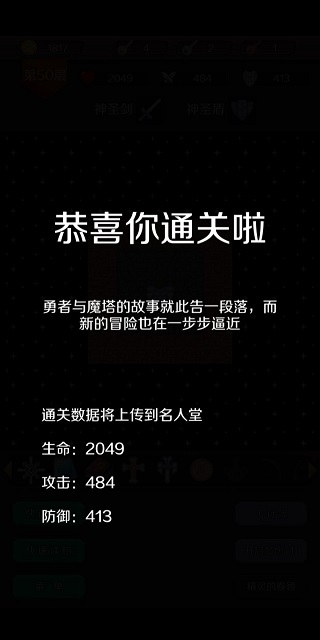49-50层：

### 游戏亮点

1、基本上每个项挑战都会在分钟内完成，所以节奏感还可以不会很慢的；
2、地牢的风格相信所有玩家都会喜欢的，很经典肯定会让所有人都爱不释手；
3、神秘任务会经常出现的，这也是其中的一个小彩蛋，充满的惊喜，值得期待。

### 更新日志

v1.7.5版本

1、新增 魔塔24层的【魔改】模式。当勇者的防御达到怪物的一定比例时，怪物变为魔攻，无视勇者的防御
2、新增 魔塔50层的【简单】【上古】模式
3、新增 魔塔50层的【成就】【帮助】
4、新增 魔塔24层的【成就】系统
5、新增查看临界
6、【经典】【上古】模式也可以使用精灵的眷顾

0条评论

最新手游